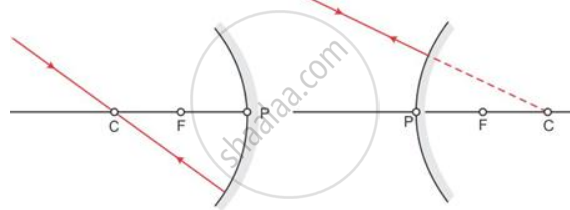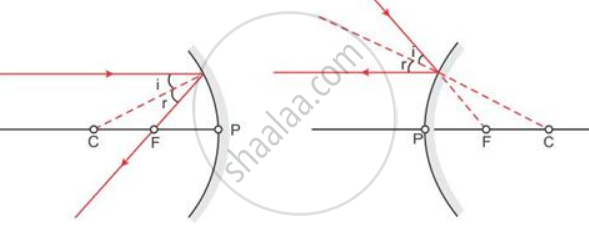# Which are the two convenient rays that are chosen to construct the image by a spherical mirror for a given object? Explain with the help of suitable ray diagrams. - Physics

Diagram

Which are the two convenient rays that are chosen to construct the image by a spherical mirror for a given object? Explain with the help of suitable ray diagrams.

#### Solution

Two convenient rays that are chosen to construct the image by a spherical mirror for a given object :

1. A ray passing through the centre of curvature: A ray of light passing through the centre of curvature of a concave mirror or a ray directed in the direction of the centre of curvature of a convex mirror is reflected back along the same path after reflection.2. A ray parallel to the principal axis: A ray of light parallel the principal axis, after reflection pass through the principal focus in case of a concave mirror or appears to diverge from it in case of a convex mirror.Concept: Spherical Mirrors
Is there an error in this question or solution?

#### APPEARS IN

Selina Concise Physics Class 8 ICSE
Chapter 5 Light Energy
Short/long Answer Question | Q 35 | Page 101